Coulombs Law and Electric Fields 26 4 to

• Slides: 9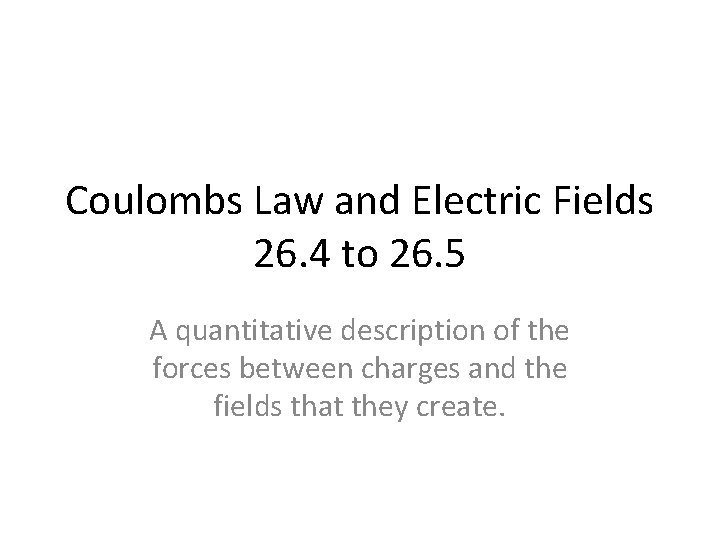Coulombs Law and Electric Fields 26. 4 to 26. 5 A quantitative description of the forces between charges and the fields that they create.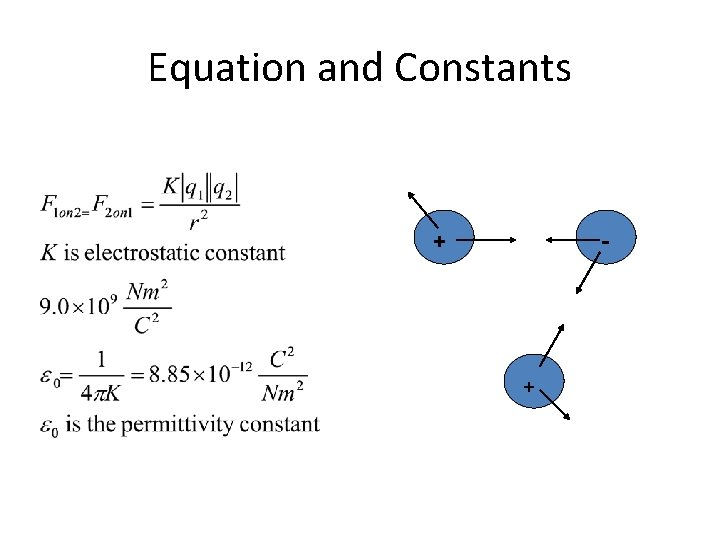Equation and Constants + - +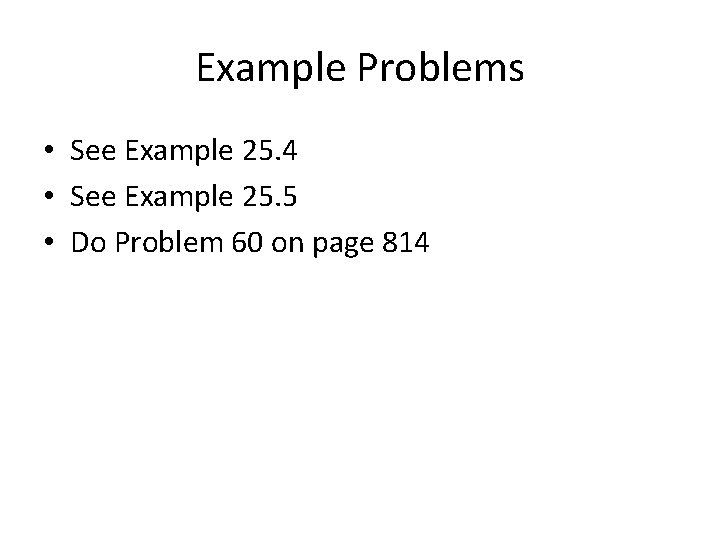Example Problems • See Example 25. 4 • See Example 25. 5 • Do Problem 60 on page 814Problem with Coulomb’s Law and Universal law of gravitation • The question arose, if a charge or mass was moved near another charge/mass does the force instantly change. There is no time dependence in either equation. Faraday introduced the field theory as an explanation.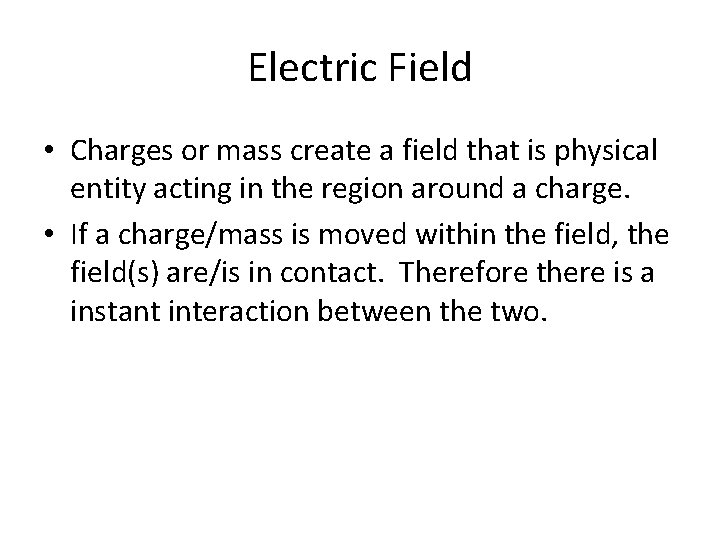Electric Field • Charges or mass create a field that is physical entity acting in the region around a charge. • If a charge/mass is moved within the field, the field(s) are/is in contact. Therefore there is a instant interaction between the two.Definition of Electric Field • The ratio of the force on a charge to the charge in the field or test charge. It is not the ratio of the force to charge creating the field.Field Model • Electric field is a vector field, meaning it exists at every location point. The diagram may only show a few of the points. • The electric field points in the same direction as the force’s direction on a positive test charge. • The electric field only depends upon the source charge. • Example – Two points charges of different quantities – http: //www. cbu. edu/~jvarrian/applets/efield 1/elefi_z. htm - – Moving Charge – http: //www. cco. caltech. edu/~phys 1/java/phys 1/Moving. Charge/Movi ng. Charge. html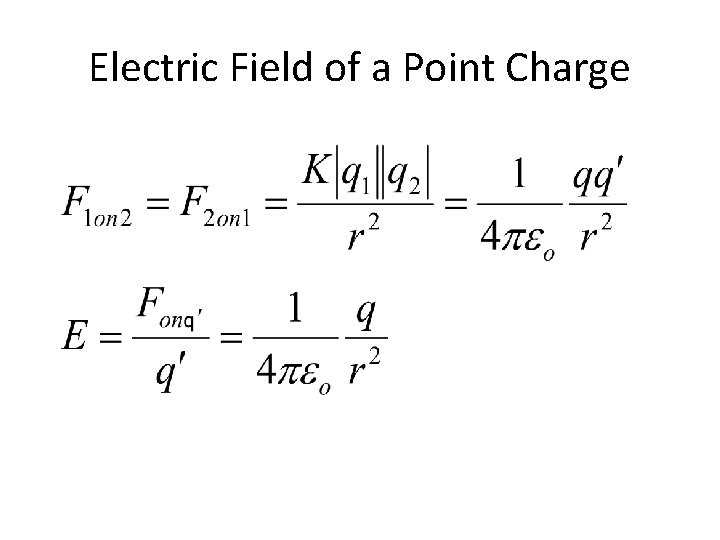Electric Field of a Point ChargeExamples of Electric Fields and Forces E Find force direction on each charge + + + + Find E direction at center